Question Pool Prisms

# Prisms - SAMAGRA Question Pool & Answers | Class 9 English Medium

Kerala Syllabus SAMAGRA SCERT SAMAGRA Question Pool for Class 9 English Medium Maths PrismsQn 1.

Find the volume of a metallic cylinder with base radius 4cm and height 10 cm?

Get Free Study Materials + 1 Week Free Trial of BrainsPrep Class 9 English Medium Tuition

Qn 2.

The length, breadth and height of a rectagular prism are respectively 10 cm, 6 cm and 20 cm. How many unit cubes ( cubes of side 1 cm) can be cut off from it ?

Get Free Study Materials + 1 Week Free Trial of BrainsPrep Class 9 English Medium Tuition

Qn 3.

A water tank is in the shape of a rectangular prism. It has length 2 m breadth 1m and height 50 cm, There

a) Find its volume

b) What is your suggestion to double its volume?

a) Volume = 1x2x.5 = 1 sq. M

b) double its

Get Free Study Materials + 1 Week Free Trial of BrainsPrep Class 9 English Medium Tuition

Qn 4.

The base radius of a cylindrical pillar with height 2m is 10 cm.

a)Find its curved face area?

b)Find the cost for painting its curved face at a rate of Rs 100/m²?

curved face area of a pillar =2 x π 10 x 200 = 4000 π sq. cm

Are of portion to bepainted = 4000 π x 10

= 40000 π sq.cm

= 4

Get Free Study Materials + 1 Week Free Trial of BrainsPrep Class 9 English Medium Tuition

Qn 5.

a) Find the third side of the right Triangle?

b) Find the base perimeter and base area of the triangle?

c) Find the volume and lateral surface area.?

a) 10 cm

b) perimeter = 24 cm

perimeter =24 sq.cm

c) Volume = 600 cu.cm

lateral surface area = 600

Get Free Study Materials + 1 Week Free Trial of BrainsPrep Class 9 English Medium Tuition

Qn 6.

The diameter of the base of a cylinder is 10 cm nad its height is 16 cm

b) Find the curved surface area

c) Find its total surface Area

Get Free Study Materials + 1 Week Free Trial of BrainsPrep Class 9 English Medium Tuition

Qn 7.

The hight of a prism, with base perimeter 20 cm and base area 30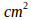is 8 cm. There

a) Find its lateral face Area

b) find its Total surface Area

a)base perimeter xheight (concept)

or

20 x 8=160 sq.cm

b) 2 x30 + 160

= 220

Get Free Study Materials + 1 Week Free Trial of BrainsPrep Class 9 English Medium Tuition

Qn 8.

The base Area of a prism is 30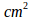and its height is 12 cm. Fin d its volume

Get Free Study Materials + 1 Week Free Trial of BrainsPrep Class 9 English Medium Tuition

Qn 9.

A solid cylinder has base diameter 10 centimeter hight 20 cm

b) How many small cylinders of base radius 2 c.m height 5 cm can be made by melting the above cylinder

Get Free Study Materials + 1 Week Free Trial of BrainsPrep Class 9 English Medium Tuition

Qn 10.

The Volumes of two cylinders are equal of the radius of the first is twice that of the second, prove that the height of the second prism is four times the height og the first

Get Free Study Materials + 1 Week Free Trial of BrainsPrep Class 9 English Medium Tuition

Qn 11.

Base areas of two prisms are in the ratio 2:3 and their heights are in the ratio 3:4

a)Find the ratio between their volumes

b) Thr volume of the first prism is 100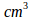find the volume of the second

Get Free Study Materials + 1 Week Free Trial of BrainsPrep Class 9 English Medium Tuition

Qn 12.

Volume of two cylinder are equal. Their base areas are in the ratio 3:4. Their

a) If the base area of the first is 3 a what is the base are aof the second

b) What is the ratio between their height?

Get Free Study Materials + 1 Week Free Trial of BrainsPrep Class 9 English Medium Tuition

Qn 13.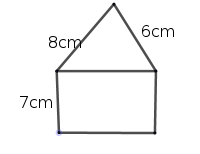In the figure the perpandicular sides of the right triangle are 6 cm and 8 cm long and the breadth of the rectangle is 7 cm Then

a) What is the length of the rectangle

b) Find the area of the figure

c) If the base of a prism having height 20 cm is as shown in the figure, find its volume

Get Free Study Materials + 1 Week Free Trial of BrainsPrep Class 9 English Medium Tuition

Qn 14.

The base edge of a regular hexagonal prism is 6 cm and its height is 10 cm

a) Find its base area and lateral surface area

b) find its total surface area

c) find its volume

Get Free Study Materials + 1 Week Free Trial of BrainsPrep Class 9 English Medium Tuition

Qn 15.

Find the quantity of soil to be removed for digging a well of radius 3 m and depth 10 m

Get Free Study Materials + 1 Week Free Trial of BrainsPrep Class 9 English Medium Tuition

Qn 16.

A wooden square prism has back edge 12 cm and height 20 cm

a) Find the diameter of the largest cylinder which can be carved out from the prism?

b) Find the volume of the cylinder.

Get Free Study Materials + 1 Week Free Trial of BrainsPrep Class 9 English Medium Tuition

Qn 17.

The length of a cylinder is 20 cm. Its curved surface area is 720 π sq. Cm

a) Find the base perimeter

b) Find the volume of the cylinder

Get Free Study Materials + 1 Week Free Trial of BrainsPrep Class 9 English Medium Tuition

Qn 18.

A plastic water tank is a place is in the shape of a rectangular prism with length 6 meter. Breadth 4 m and depth 3 m. There is water at a height of 1 m in this tank

a) How many litter of water in it

b) How many litters of water needed to fill the tank

Get Free Study Materials + 1 Week Free Trial of BrainsPrep Class 9 English Medium Tuition

Qn 19.

The base of a prism is an equilateral triangle having perimeter 18 cm Its height is 10 cm Then

a) Find the length of one side of the base

b) Find the volume of the prism

Get Free Study Materials + 1 Week Free Trial of BrainsPrep Class 9 English Medium Tuition

Qn 20.

The diagram shows a prism which is cut and laid flat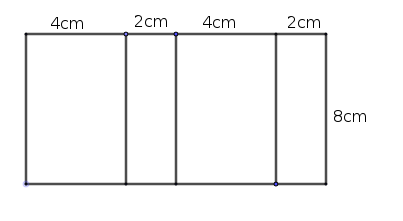a) Write the suitable name of the base of the prisms

b) Find the perimeter and Area of the base.

c) Find the Volume of the prism.

a) rectangle

b) Perimeter 2(4x2) = 16

Area 4x2 = 8

c) Volume = 8x8 = 64 cubic

Get Free Study Materials + 1 Week Free Trial of BrainsPrep Class 9 English Medium Tuition

Qn 21.

A wooden peace in the shape of square prism has the base area 900 sq cm and height 50 cm then

a) What is its volume?

b) Find the maximum number of planks of length 50cm, breadth 30 cm, and thickness 5 cm that can be cut out from the wooden piece

c) If the length, bredth and thickness of one plank is 50 cm ,20cm, and 5 cm then find the maximum number of planks that can be cut out from the wooden piece